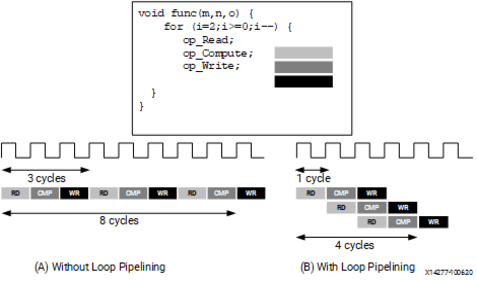# Pipelining Loops - 2022.2 English

## Vitis High-Level Synthesis User Guide (UG1399)

Document ID
UG1399
Release Date
2022-12-07
Version
2022.2 English

Pipelining loops permits starting the next iteration of a loop before the previous iteration finishes, enabling portions of the loop to overlap in execution. By default, every iteration of a loop only starts when the previous iteration has finished. In the loop example below, a single iteration of the loop adds two variables and stores the result in a third variable. Assume that in hardware this loop takes three cycles to finish one iteration. Also, assume that the loop variable `len` is 20, that is, the `vadd` loop runs for 20 iterations in the kernel. Therefore, it requires a total of 60 clock cycles (20 iterations * 3 cycles) to complete all the operations of this loop.

``````vadd: for(int i = 0; i < len; i++) {
c[i] = a[i] + b[i];
}``````
Tip: It is good practice to always label a loop as shown in the example above (`vadd:…`). This practice helps with debugging the design in Vitis HLS. Sometimes the unused labels generate warnings during compilation, which can be safely ignored.

Pipelining the loop allows subsequent iterations of the loop to overlap and run concurrently. Pipelining a loop can be enabled by adding the pragma HLS pipeline inside the body of the loop as shown below:

``````vadd: for(int i = 0; i < len; i++) {
#pragma HLS PIPELINE
c[i] = a[i] + b[i];
}``````
Tip: Vitis HLS automatically pipelines loops with 64 iterations or more. This feature can be changed or disabled using the ```config_compile -pipeline_loops``` command.

The number of cycles it takes to start the next iteration of a loop is called the Initiation Interval (II) of the pipelined loop. So II = 2 means the next iteration of a loop starts two cycles after the current iteration. An II = 1 is the ideal case, where each iteration of the loop starts in the very next cycle. When you use pragma HLS pipeline, you can specify the II for the compiler to achieve. If a target II is not specified, the compiler will try to achieve II=1 by default.

The following figure illustrates the difference in execution between pipelined and non-pipelined loops. In this figure, (A) shows the default sequential operation where there are three clock cycles between each input read (II = 3), and it requires eight clock cycles before the last output write is performed.

Figure 1. Loop PipeliningIn the pipelined version of the loop shown in (B), a new input sample is read every cycle (II = 1) and the final output is written after only four clock cycles: substantially improving both the II and latency while using the same hardware resources.

Important: Pipelining a loop causes any loops nested inside the pipelined loop to get automatically unrolled.

If there are data dependencies inside a loop, it might not be possible to achieve II = 1, and a larger initiation interval might be the result. Loop dependencies are data dependencies that may constrain the optimization of loops, typically pipelining. They can be within a single iteration of a loop and or between different iterations of a loop. The easiest way to understand loop dependencies is to examine an extreme example. In the following example, the result of the loop is used as the loop continuation or exit condition. Each iteration of the loop must finish before the next can start.

``````Minim_Loop: while (a != b) {
if (a > b)a -= b;
else b -= a;
}``````

The `Minim_Loop` loop in the example above cannot be pipelined because the next iteration of the loop cannot begin until the previous iteration ends. Not all loop dependencies are as extreme as this, but the example highlights that some operations cannot begin until some other operation has been completed. The solution is to try to ensure that the initial operation is performed as early as possible.

Loop dependencies can occur with any and all types of data. They are particularly common when using arrays.